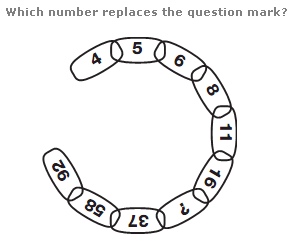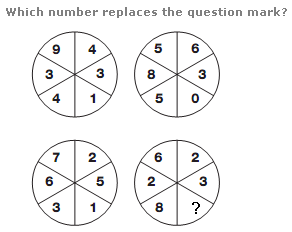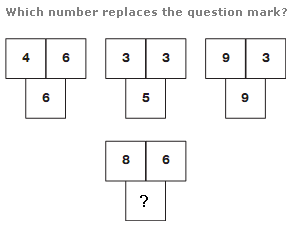# Puzzles - Number puzzles

### Exercise :: Number puzzlesAnswer : 24 Explanation : Working clockwise around the diagram, add the first two numbers together and subtract 3 to give the next number along.Answer : 3 Explanation : In each circle, the sum of the numbers in the left hand half of the circle equals double that of the numbers in the right hand half of the circle.Answer : 11 Explanation : Starting with the diagram in the top left, and moving to the right, ending up with the bottom diagram, average the values in the top two boxes and put the result in the lower box, adding 1 for the first diagram, 2 for the next, then 3, then 4.